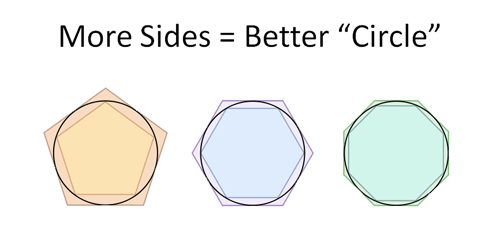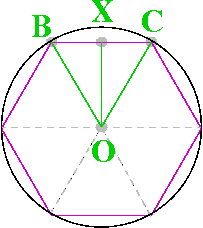## Proof of π and circumference of circle

0 / 4456## What is pi ?

pi is the the sixteenth letter of the Greek alphabet ( Π, π ), transliterated as ‘pi’. It’s approximate value is 3.14159265359 or 22/7.

In this blog , we try to find out the proof for the approximation of the value of pi (Π).

To approximate the value of pi , we will use the same kinda approach as in the previous post for the proof of area of circle.

## Proof of Π(pi)

Consider the below diagrams:Figure I – pi value approximationFigure II – hexagon in circle

We know that the measure of angle of a polygon is 180(n-2)/n, where n is the number of sides of polygon. Also, when we look at figure II above,

we know that for a polygon (hexagon in above case), ∠OBC ≅ ∠OCB and it’s half of the interior angle of polygon because all internal triangles within a polygon are congruent and the radius bisects each of the interior angles of a polygon, thus we have

m∠OBC = m∠OCB =  1/2 *(measure of interior angle of polygon) =Similarly we have ∠BOX ≅ ∠XOC =1/2*m∠BOC –————(a)

Now  m∠BOC = 180-2*m∠OBC = 180-2*90*(n-2)/n = 360/n—–(b)

From (a) and (b) we have m∠BOX = m∠XOC = 1/2*360/n = 180/nFrom the above figures I and III, we can clearly see that the perimeter of circle lies between the 2 polygons, as it’s sandwiched between them. Similarly, side of inner triangle is 2RSin30°

Thus, collating all results we have for a hexagon we have

6*2RSin30° < Circumference of circle < 6*2RTan30°

further solving this, we have:-

6*2RSin30° < 2ΠR <  6*2RTan30°

=>6Sin30° < Π <6 Tan30°=> 3 < Π < 3.46.

By increasing the number of sides of polygon this estimate can be improved and as a generic formula we have

for a n-gon   n*Sin(180/n) < Π  < n*Tan(180/n) —————-(c)In equation (c) above, say, for eg, we consider polygon with 180 sides and substituting n=180 , we get

180*Sin(180/180) < Π < 180*Tan(180/180)

=> 180*Sin1° < Π < 180*Tan1°

=> 3.14143315871 < Π < 3.14191168708.

As, we go on increasing the value of n, the closer we get to the approximation of pi. See the pi approximation table below:-## Proof of circumference/perimeter of circle

Examining the proof of pi carefully, we can say that the circumference of circle lies between the circumference of the 2 polygons as seen in the above diagrams i.e 2R*n*Sin(180/n) and 2R*Tan(180/n) where n = number of sides of polygon and R is the radius of circle.

Also pi lies between n*Sin(180/n) and n*Tan(180/n) as proved above and hence we have circumference of circle = 2πR.